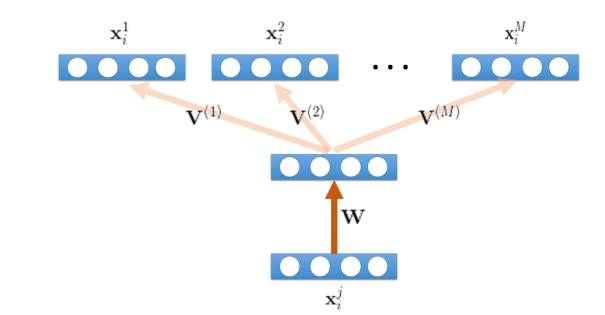# Multi-task autoencoder loss stuck and not converging

Hi @ptrblck , I am trying to implement a multi-task loss function where I have one encoder and multiple decoders. The input is from multiple domains and it tries to jointly achieve good reconstruction of all source data given a particular segment of train data.In order to achieve it, all the samples from different domains are concatenated together like this. Here 1-M are the different domains and l is the replicated data from the lth domain.The code is below:

import torch
import torch.nn as nn
from dataset import Dataset
from autoencoder import Autoencoder
import numpy as np

device = torch.device(“cuda:0” if torch.cuda.is_available() else “cpu”)

num_epochs = 500
batch_size = 27
learning_rate = 0.005
feats = 29
domains = 3
latent_dims = 10

model = Autoencoder().to(device)
criterion = nn.MSELoss()

``````def __init__(self):
super().__init__()

self.encoder = nn.Sequential(
nn.Linear(feats, latent_dims),
nn.ReLU(),
# nn.Linear(4, 3)
)
self.decoders = nn.ModuleList()
for _ in range(batch_size):
self.decoders.append(nn.Linear(10, feats))

self.encoder_hidden_layer = nn.Linear(
in_features=feats, out_features=20
)
self.encoder_output_layer = nn.Linear(
in_features=20, out_features=10
)

def forward(self, inputs): #inputs--> batch_size

activation = self.encoder_hidden_layer(inputs)
activation = torch.relu(activation)
code = self.encoder_output_layer(activation)
code = torch.relu(code)
#z.append(code)

outs = []
for idx, dec in enumerate(self.decoders):
outs.append(dec(code[idx]))
outs = torch.stack(outs)
return outs
``````

def train_AE():
dataset = Dataset()

``````for epoch in range(100):
train_loss = 0.0

input = input.float().to(device)

#print('input.shape = ', input.shape)  # 128 * 256
dom_out = out.float().to(device) ## original data # 128 * 256
#print('dom_out.shape = ', dom_out.shape) # 128 * 256

#print('predicted output.shape = ', output.shape) # 128 * 256 -- reconstructed values

loss = criterion(output, dom_out)

loss.backward()

train_loss += loss.item()

print('===> Loss: ', train_loss / len(dataloader))
``````

train_AE()

The dataset generator is as below:

def construct_pair(X_list): # 3 * 10000 * 29

``````n_dom = len(X_list) # 3 * 10000 * 29
#print("Xin shape BEFORE virtual stack:", X_list.shape)

X_in = np.vstack(X_list)  ## 30000 * 29
print("Xin SHAPE TRAIN DATA", X_in.shape)

X_outs = [] # ground truth data
for i in range(0, n_dom): # for each domain
X = X_list[i]  # take first domain 1 * 10000 * 29

print("X shape:", X.shape) ## X shape: 1 * 10000 * 29

Z_list = []
for j in range(0, n_dom):
Z_list.append(X) # make 3 (num of domains) copies of the same 1000 samples. 3 * 10000 * 29

Z = np.vstack(Z_list) # Z shape: 30000 * 29
print("Z shape:", Z.shape)

X_outs.append(Z) #  3 * 30000 * 29 ## same samples repeated to nos of domains.

print("XOUT SHAPE ", len(X_outs))
return X_in, X_outs
``````

Blockquote

My loss value is stuck and loss is not converging. Can you please give some hints on what am I doing wrong here? Thanks a lot!

Hi, @ptrblck , can you plz provide me some hints here? Thanks!

I’ve responded to your cross-post already and mentioned that I wouldn’t know which approach works best for your use case (here). I would thus recommend avoiding tagging specific users as it might discourage others to post a valid answer.
Generally, you could try to overfit a small dataset (e.g. just 10 samples) and make sure your model is able to learn these samples (almost) perfectly. If that’s not the case your model architecture or overall training could have other errors or might just not be suitable for the task.

hi , I am sorry for this tagging mistake, and thanks for your suggestion. I will keep this open now so that I can get some hints from others as well.

Hi All, the idea I am trying to implement is from this paper “Domain Generalization for Object Recognition with Multi-task Autoencoders”- https://arxiv.org/pdf/1508.07680.pdf.
I have a confusion about the decoder architecture - do I need to split the encoder output into M parts and pass it through the decoder with equal inputs? Shall i keep all decoder architectures the same here? Kindly provide some input here .